# Difference between revisions of "Calibrating Analog Sensors"

Analog sensors work by converting some real world parameter such as temperature or pressure or weight into an analog voltage that is measurable by your computer. Once the computer has this voltage you can perform some calculation to convert that back into a reading in a unit you can work with (°C for example). Many sensors will include a formula in their documentation that describes this relationship between voltage output and the measured quantity. This is a reasonable solution when the part to part variance in a product line is very low but it is very common for part to part variance to be high enough that a formula that is exactly correct for one sensor to be inaccurate for another sensor of the same model by several percent. To solve this problem we generally recommend that you generate your own formula for each individual sensor you are using in your project. This process, called calibration, is a very important step to achieve maximum accuracy.

## How to calibrate a sensor

In the general sense, the output voltage of the sensor is related to the measured parameter via some function f(x). In order to determine what f(x) for a given sensor is, we first have to obtain some data. As an example, let's consider the case of a load cell. Load cells are a sensor for measuring weight and are one of the most common types of sensor you might find yourself needing to calibrate. Here I have assembled a simple scale with a load cell rated for a maximum weight of 5kg. In practice you would install the sensor in whatever system you intend to use it in and calibrate in situ.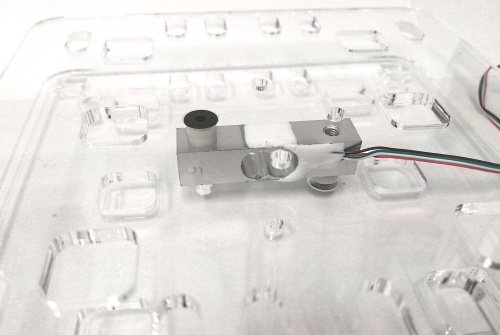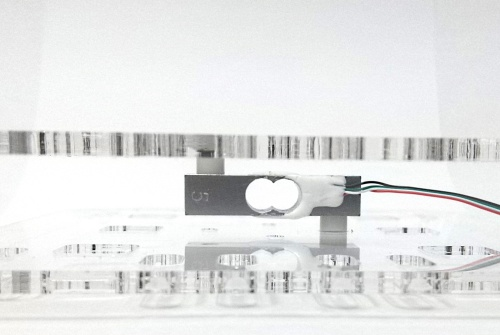To begin calibration I will take a number of data points with objects that I know the weight of already (I weight some products we sell with our shipping scale but you would ideally use proper weights). The more data points you take, the more accurate your final formula will be but this can be time consuming and impractical for a large number of sensors. Looking at the graph of the data however, we can see that the data appears to be very linear.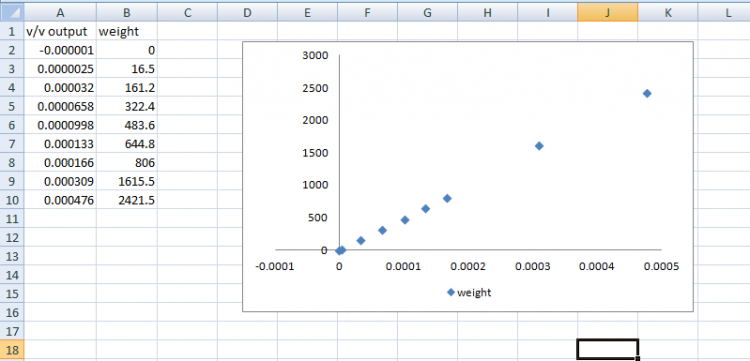This leads us to a major shortcut in calibrating a sensor: 2-point calibration. Whenever possible analog sensors are designed to have a linear response like this. It's a massive simplification since in linear systems we really only need to take 2 data points in order to have a reasonably accurate trendline as I will now demonstrate. Since we have all the data already, let's determine the equation that best fits this data. In a spreadsheet application like Excel this is an easy, automated process.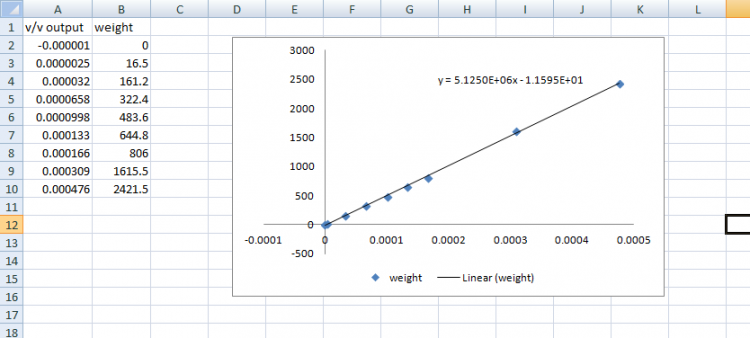I have generated this trendline use Excel's built in linear regression function and you can see that the line fits the data quite closely. So we now have our function f(x):

${\text{f(x)}}=5.125E6{\text{x}}-1.1595$

Where x is our measured V/V from the load cell and f(x) is the calculated weight. These measurements are in grams but notice that there are no units in my data points or the resultant graph or trendline. This is one of the nice things about the process, it works for any unit. The formula we end up with will convert our measured voltage into the same unit that we use to take the data points initially be it pounds or grams or tons. As a quick example to prove this has actually worked, let's take a new object we know the weight of and see that the formula spits out the right number. These 2 motors have a combined weight of 1810g: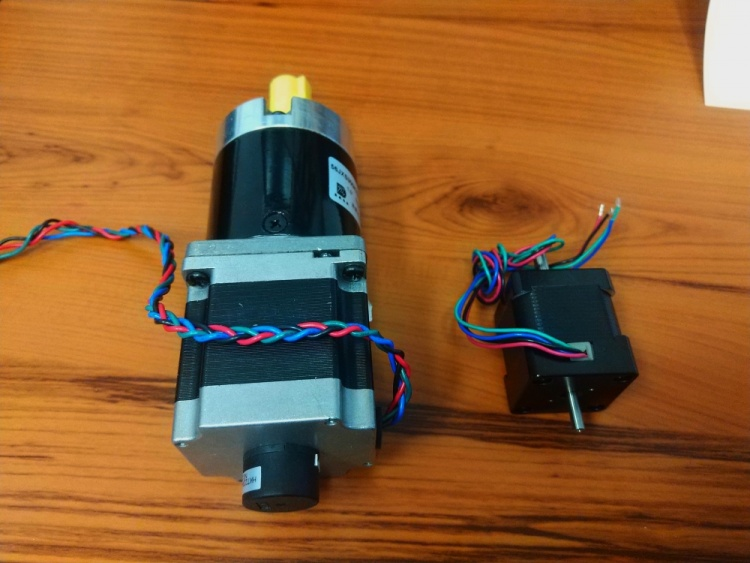When applied to the load cell I measure 0.00035V/V: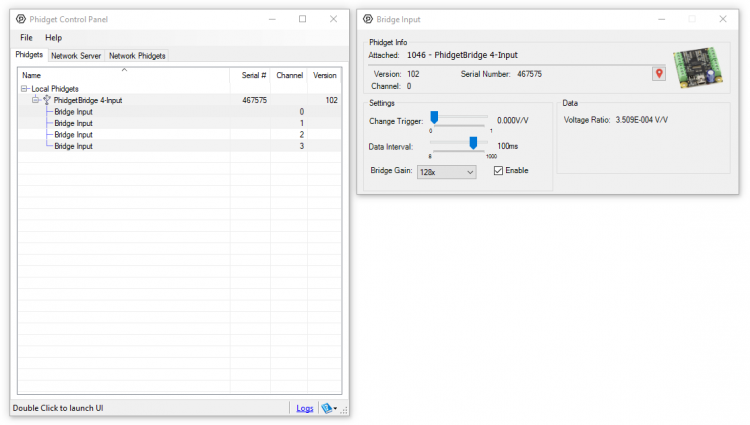According to our formula this means it detects a weight of 5.125E6*0.00035 - 1.1595 = 1792.6g which is within 20g of our expected weight which for a 5kg load cell is an accuracy of about 0.4%. Not too bad. We could improve this with more calibration points, more accurate weights of the measured objects, , by using more of the load cell's range (we only went up to about 2.5kg here), or more decimal points on the measurements we took from the device.

Now to show that we can make this a bit simpler, let's try a 2-point calibration for the same load cell. I will use the same data collected previously, I will just only use the highest and lowest points. When doing a 2-point calibration (90% of the time you calibrate a sensor this is what you will be doing) you generally want to use 1 point from rest (0 weight in this case) and 1 point from as close to the maximum of the range as you can (5kg in this case though I will be using the point from about 2.5kg from before). We could use Excel again to do the calculation for us but it's so much easier this time that I will just do it by hand. We have our 2 points:

 :${\text{(V/V}}(x1)=-0.000001{\text{,Weight}}(y1)=0{\text{)}}$ :${\text{(V/V}}(x2)=0.000476{\text{,Weight}}(y2)=2421.5{\text{)}}$
${\text{Slope}}=y2-y1/x2-x1=5.0765E6$

Then we can try a test case to determine our b parameters for a y=mx+b style linear equation using one of our data points:

$y2=m*x2+b=2421.5=5.0765E6*0.000476+b$

So b = 5.07652

Which gives:

${\text{f(x)}}=5.0765E6x+5.07652$

Not quite exactly the same as our initial formula but let's have a look at the results. With this new formula let's try the same conversion as before with a measurement of 0.00035V/V. This gives 5.0765E6*0.00035 + 5.07652 = 1781.9g which is a fairly comparable result for much less time spent taking measurements. The greater the non-linearity in our sensor the more unreliable this will become but for most sensors the non-linearity will be lower than the overall accuracy of the sensor meaning you can effectively ignore effects from non-linearity.